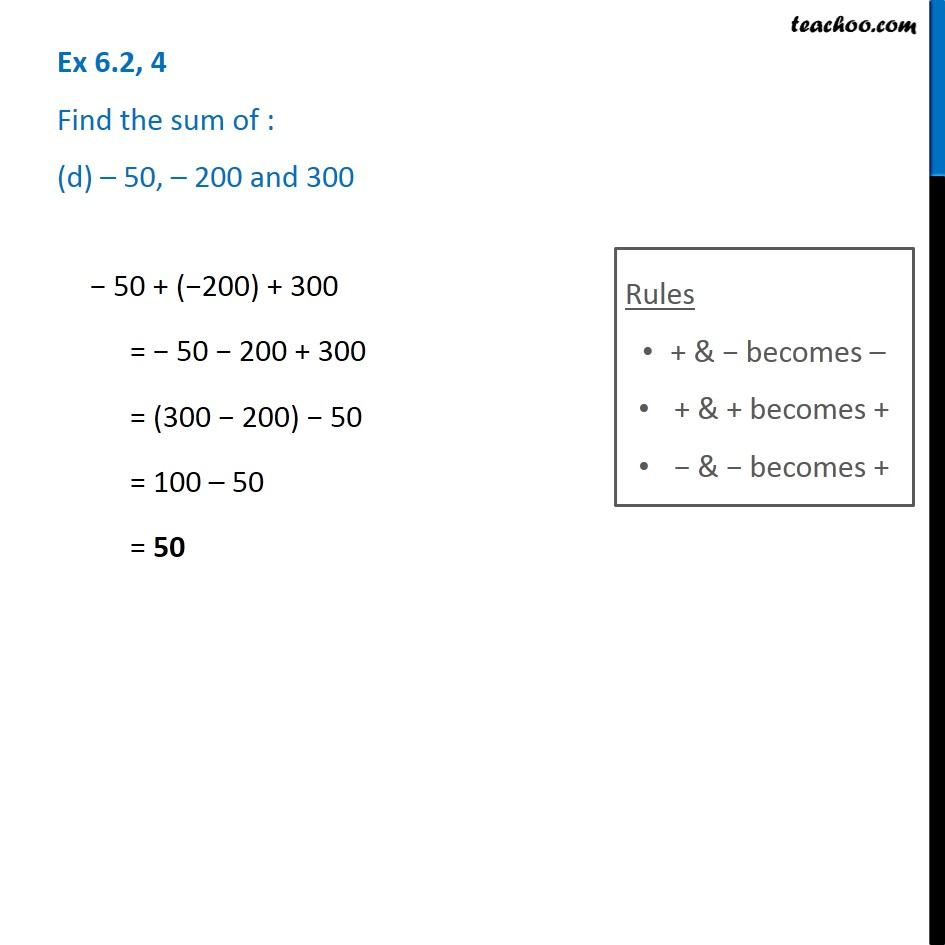1. Chapter 6 Class 6 Integers
2. Serial order wise
3. Ex 6.2

Transcript

Ex 6.2, 4 Find the sum of : (d) – 50, – 200 and 300 − 50 + (−200) + 300 = − 50 − 200 + 300 = (300 − 200) − 50 = 100 – 50 = 50

Ex 6.2

Chapter 6 Class 6 Integers
Serial order wise